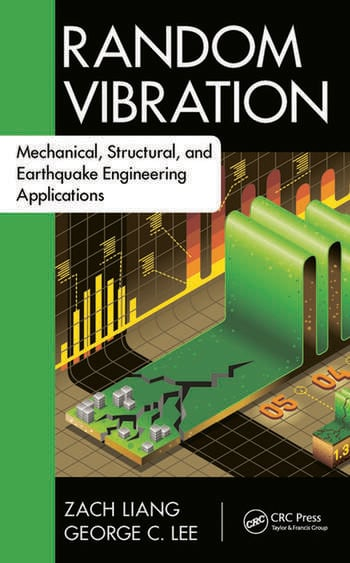Random Vibration: Mechanical, Structural, and Earthquake Engineering Applications

1st Edition

Zach Liang, George C. Lee

CRC Press
Published April 14, 2015
Textbook - 668 Pages - 192 B/W Illustrations
ISBN 9781498702348 - CAT# K24606

For Instructors Request Inspection Copy

USD\$155.00

FREE Standard Shipping!

Preview

Summary

Focuses on the Basic Methodologies Needed to Handle Random Processes

After determining that most textbooks on random vibrations are mathematically intensive and often too difficult for students to fully digest in a single course, the authors of Random Vibration: Mechanical, Structural, and Earthquake Engineering Applications decided to revise the current standard. This text incorporates more than 20 years of research on formulating bridge design limit states. Utilizing the authors’ experience in formulating real-world failure probability-based engineering design criteria, and their discovery of relevant examples using the basic ideas and principles of random processes, the text effectively helps students readily grasp the essential concepts. It eliminates the rigorous math-intensive logic training applied in the past, greatly reduces the random process aspect, and works to change a knowledge-based course approach into a methodology-based course approach. This approach underlies the book throughout, and students are taught the fundamental methodologies of accounting for random data and random processes as well as how to apply them in engineering practice.

Gain a Deeper Understanding of the Randomness in Sequences

Presented in four sections, the material discusses the scope of random processes, provides an overview of random processes, highlights random vibrations, and details the application of the methodology. Relevant engineering examples, included throughout the text, equip readers with the ability to make measurements and observations, understand basic steps, validate the accuracy of dynamic analyses, and master and apply newly developed knowledge in random vibrations and corresponding system reliabilities.

Comprising 11 Chapters, this text:

• Reviews the theory of probability and applies it from an engineering perspective
• Introduces basic concepts and formulas to prepare for discussions of random processes
• Emphasizes the essence of probability as the chance of occurrence in sample space
• Covers two important issues in engineering practice, the uncertainty of data and the probability of failure
• Explores the random processes in the time domain
• Explains the nature of time-varying variables by joint PDF through the Kolmogorov extension
• Examines random processes in the frequency domain
• Discusses several basic and useful models of random processes
• Presents a new set of statistics for random processes
• Employs an approach to present important processes within the context of practical engineering problems
• Includes the generality of dealing with randomness and the difference between random variables and processes
• Focuses on the topic of vibration problems
• Addresses the basic parameters of linear single-degree-of-freedom (SDOF) systems
• Stresses a new method of random process referred to as time series
• Details linear multi-degree-of-freedom (MDOF) systems
• Describes the statistical analyses of direct approach based on model decoupling of proportionally and nonproportionally-damped systems
• Provides materials on the applications of random processes and vibration
• Discusses statistical studies on random data and model identifications
• Describes the nonlinear phenomena and the general approach of linearization
• Highlights a special method of Monte Carlo simulation, and more

Random Vibration: Mechanical, Structural, and Earthquake Engineering Applications effectively integrates the basic ideas, concepts, principles, and theories of random processes. This enables students to understand the basic methodology and establish their own logic to systematically handle the issues facing the theory and application of random vibrations.

Instructors

We provide complimentary e-inspection copies of primary textbooks to instructors considering our books for course adoption.